# Symmetrical Components

In 1918, Dr.C.L. Fortescue, an American scientist, showed that the theory of symmetrical components for any unbalanced system of 3 phase currents or voltages may be regarded as being composed of three separate sets of balanced vector viz.
• a balanced system of three phase currents having positive phase sequence. These are called positive phase sequence components.
• a balanced system of three phase currents having the opposite or negative phase sequence. These are called negative phase sequence components.
• a system of three currents equal in magnitude and having zero phase displacement. These are called zero phase sequence components.
The positive, negative and zero phase sequence components are called the symmetrical components of the original unbalanced system. The word ‘symmetrical’ is appropriate because the unbalanced 3 phase system has been resolved into three sets of balanced or symmetrical components. The subscripts 1, 2 and 0 are generally used to indicate positive, negative and zero phase sequence components respectively. For instance,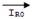indicates the zero phase sequence components of the current in the Red phase. Similarly,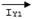implies the positive phase sequence component of current in the Yellow phase.

# Illustration of Symmetrical Components: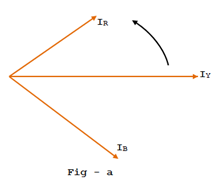Now let us apply the symmetrical components theory to an unbalanced three phase system. Suppose a symmetrical fault occurs on a 3-phase system having phase sequence RYB. According to symmetrical components theory the resulting unbalanced current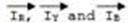(see fig-a) can be resolved into a balanced system of 3-phase currents,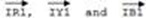having positive sequence - RYB as shown in the fig-i. These are the positive phase sequence components.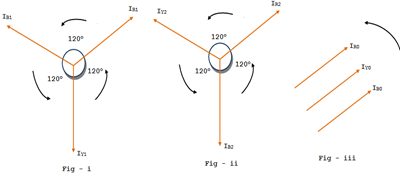##### Symmetrical Components – Phase Sequence
1. a balance system of 3-phase currents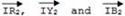having negative phase sequence  - RBY as shown in fig-ii. These are the negative phase sequence components.
2. a system of three currents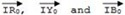equal in magnitude with zero phase sequence components.
The current in any phase is equal to the vector sum of positive, negative and zero phase sequence currents in that phase as shown in fig. below.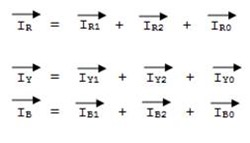The following points may be noted:
• The positive phase sequence currents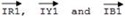, negative phase sequence currents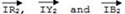and zero phase sequence currents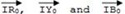separately form balanced system of currents. Hence, they are called symmetrical components of the unbalanced system.
• The symmetrical components theory applies equally to 3-phase currents and voltages both for phase and line values.
• The symmetrical components do not have separate existence. They are only mathematical components unbalanced currents or voltages which actually flow in the system.
• In a balanced 3-phase system, negative and zero phase sequence currents are zero.1 CommentsComments

#### Read Comment Policy We have Zero Tolerance to Spam. Chessy Comments and Comments with Links will be deleted immediately upon our review.

1.https://www.ace4sure.com/1z0-1060-questions.html is the website that deals in preparation material for the exam for many years. According to my exposure and research, this is the right platform where you can get exact exam dumps.